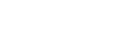# How to see last login timestamp for users?

I’m trying to identify and disable unused accounts for my XWiki instance. Confluence had a sortable “last login timestamp” column in the user list that administrators could use to filter users who do not appear to be using their Confluence accounts anymore. Does XWiki have a similar way to determine when was the last time that a user logged in?

``````{{velocity wiki="true"}}

##set(\$hours = \$util.parseInt("\$context.macro.params.hours"))
#set (\$hours = 2*7*24)
#set(\$period = \$xwiki.jodatime.dateTime.minusHours(\$hours))
#set(\$formatter = \$xwiki.jodatime.getDateTimeFormatterForPattern("yyyy-MM-dd HH:mm:ss.S"))
#set(\$date = \$formatter.print(\$period))
#set(\$now = \$xwiki.jodatime.dateTime)

#set (\$sql = "select distinct(stats.name),max(stats.endDate) from VisitStats as stats where stats.name <> 'XWiki.XWikiGuest' and stats.endDate > '\$date' group by stats.name order by max(stats.endDate) desc")
#set (\$results = \$xwiki.search(\$sql))
#set (\$count = 1)
#foreach (\$result in \$results)
#set (\$lastupdatedate = \$formatter.parseDateTime(\$result.toString()))
#set (\$diff = (\$now.millis - \$lastupdatedate.millis) / 1000)
#set (\$diffJ = (\$diff / 60 / 60 / 24))
#set (\$diffH = (\$diff / 60 / 60) - (\$diffJ * 24) )
#set (\$diffM = (\$diff / 60) - (\$diffH * 60))
#set (\$diffStr = "")
#if (\$diffJ == 0 && \$diffH == 0 && \$diffM == 0)
#set (\$diffStr = "now")
#else
#if (\$diffJ != 0)
#set (\$diffStr = "\${diffJ}d")
#end
#if (\$diffH != 0 || \$diffJ != 0)
#if (\$diffStr != "")
#set (\$diffStr = "\$diffStr, ")
#end
#set (\$diffStr = "\${diffStr}\${diffH}h")
#end
#if (\$diffJ == 0 && \$diffM != 0)
#if (\$diffStr != "")
#set (\$diffStr = "\$diffStr, ")
#end
#set (\$diffStr = "\${diffStr}\${diffM}min")
#end
#set (\$diffStr = "\${diffStr} ago")
#end
| [[\$result]] | \$xwiki.getUserName(\$result,false)  | (\${diffStr})
#set (\$count = \$count + 1)
#end
{{/velocity}}
``````

It generates a sorted table of xwiki visits the last 14 days (\$hours).

1 Like